# Calculate the frequency counts of each unique value of a Pandas series

Let us see how to find the frequency counts of each unique value of a Pandas series. We will use the value_counts() function to perform this task.

Example 1 :

 `# importing the module ` `import` `pandas as pd ` ` `  `# creating the series ` `s ``=` `pd.Series(data ``=` `[``2``, ``3``, ``4``, ``5``, ``5``, ``6``,  ` `                      ``7``, ``8``, ``9``, ``5``, ``3``]) ` ` `  `# displaying the series ` `print``(s) ` ` `  `# finding the unique count ` `print``(s.value_counts()) `

Output :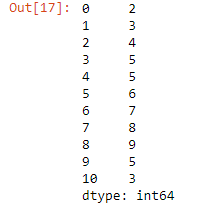Output: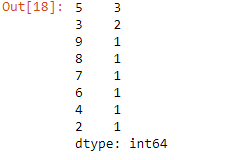Example 2 :

 `# importing the module ` `import` `pandas as pd ` ` `  `# creating the series ` `s ``=` `pd.Series(np.take(``list``(``'0123456789'``),  ` `              ``np.random.randint(``10``, size ``=` `40``))) ` ` `  `# displaying the series ` `print``(s) ` ` `  `# finding the unique count ` `s.value_counts() `

Output :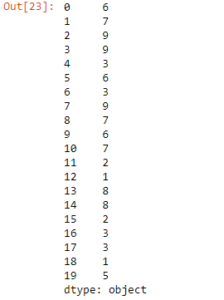Output: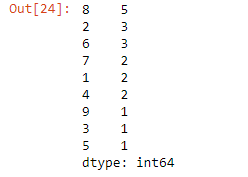Example 3 :

 `# importing pandas as pd  ` `import` `pandas as pd  ` `   `  `# creating the Series  ` `sr ``=` `pd.Series([``'Mumbai'``, ``'Pune'``, ``'Agra'``, ``'Pune'``,  ` `                ``'Goa'``, ``'Shimla'``, ``'Goa'``, ``'Pune'``])  ` ` `  `# displaying the series  ` `print``(sr)  ` ` `  `# finding the unique count ` `sr.value_counts() `

Output :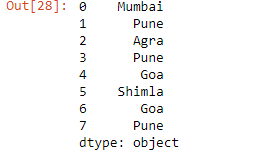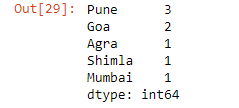Attention geek! Strengthen your foundations with the Python Programming Foundation Course and learn the basics.

To begin with, your interview preparations Enhance your Data Structures concepts with the Python DS Course.

My Personal Notes arrow_drop_upCheck out this Author's contributed articles.

If you like GeeksforGeeks and would like to contribute, you can also write an article using contribute.geeksforgeeks.org or mail your article to contribute@geeksforgeeks.org. See your article appearing on the GeeksforGeeks main page and help other Geeks.

Please Improve this article if you find anything incorrect by clicking on the "Improve Article" button below.

Article Tags :

Be the First to upvote.

Please write to us at contribute@geeksforgeeks.org to report any issue with the above content.